September 30, 2023

# Class 8 Maths Chapter 1 (Rational numbers) Exercise 1.1

## Class 8 maths chapter 1 exercise 1.2 solutions

NCERT maths class 8 chapter 1  (Exercise 1.2)

1. Represent these numbers on the number line. (i)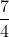(ii)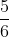Sol.  (i) To represent  the number line may be divided into four equal parts as shown  fig 1.  We use the number to name the first point of this division. The second point of division will be labelled , the third point , and so on . The point A will represent .

(ii)  To represent  the number line may be divided into six equal parts as shown  fig 2.  We use the number to name the first point of this division. The second point of division will be labelled , the third point , and so on . The point B will represent  .

2. Represent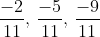on the number line.

Sol.  Point A is  ,  point B is and point C is .

3. Write five rational numbers which are smaller than 2.

Sol. Five rational numbers smaller than 2 are

4. Find ten rational numbers between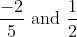.

Sol.  We first convert to rational numbers with the same denominators.

and

Thus we have  between .

You can take any ten of these. In fact , you get countless rational numbers between any two given rational numbers.

5. Find five rational numbers between (i)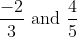(ii)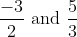(iii)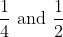Sol. (i) can be written as   and   as .

Thus we have   between    and .

You can take any five of these.

(ii) can be written as   and   as  .

Thus we have   between    and  .

You can take any five of these.

(iii) can be written as   and   as  .

Thus we have are five rational numbers   between    and  .

6. Write five rational numbers greater than –2.

Sol. The five rational numbers greater than -2  are   .

7. Find ten rational numbers between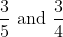.

Sol. We first convert  to rational numbers with the same denominators.

and

Thus we have   between .

You can take any ten of these. In fact , you get countless rational numbers between any two given rational numbers.#### Bina singh

View all posts by Bina singh →
ssc chsl 2023 tier 1 cut off NIRF Rankings 2023 : Top 10 Engineering colleges in India CBSE Compartment Exam 2023 Application Form SSC CGL 2023 Notification: Important Dates UPSC Recruitment 2023